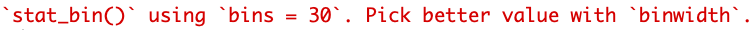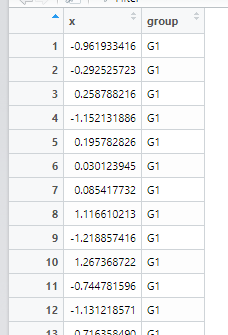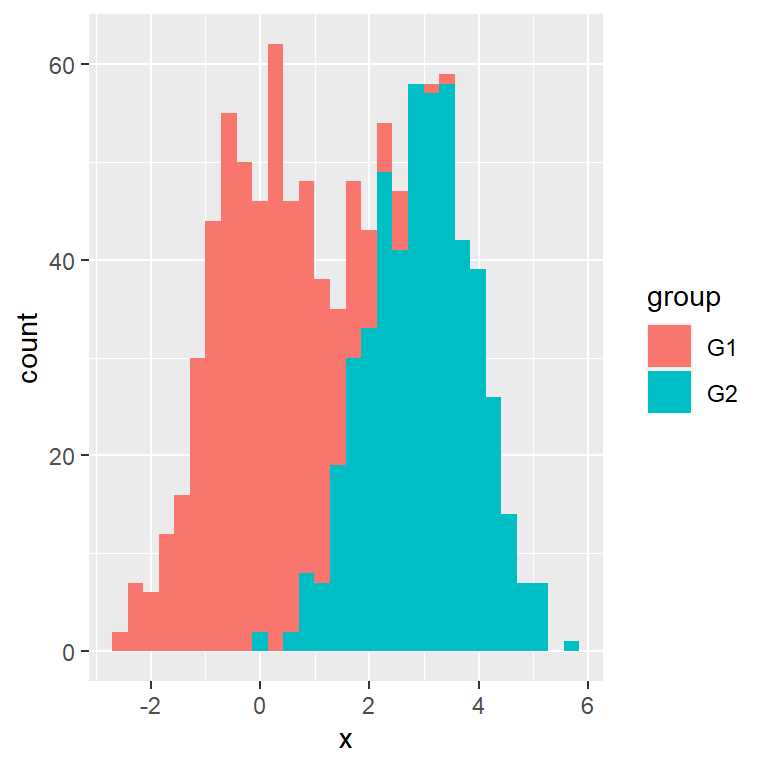• R语言ggplot2可视化：ggplot2可视化直方图（histogram）并在直方图的顶部外侧（top upper）或者直方图内部...
2021-12-13 22:53:28

# R语言ggplot2可视化：ggplot2可视化直方图（histogram）并在直方图的顶部外侧（top upper）或者直方图内部添加数值标签

目录机器学习 数据挖掘 人工智能 自然语言处理
更多相关内容
• ggplot2直方图最基本的代码为： ggplot(data=travel)+geom_histogram(aes(x=sale)) 这里面travel是data frame，sale是我要画直方图的数据。 这个时候会出现warning: 这说明他默认了直方图中“柱”的个数，但是...

# 1.基本代码

用ggplot2画直方图最基本的代码为：

ggplot(data=travel)+geom_histogram(aes(x=sale))


这里面travel是data frame，sale是我要画直方图的数据。
这个时候会出现warning:这说明他默认了直方图中“柱”的个数，但是其实我是可以自己设置的。既可以通过bin的个数设置，也可以通过bin的宽度设置：

ggplot(data=travel)+geom_histogram(aes(x=sale),bins=20)

ggplot(data=travel)+geom_histogram(aes(x=sale),binwidth=20)


# 2.对数变换

我们常常遇到的数据都是偏态的，直方图非常不美观：这时候可以通过对数变换解决：

ggplot(data=travel)+geom_histogram(aes(x=log(sale)))这里横坐标的值就变成了对数值，如果还希望图片中显示对应的没有对数变换过的值，可以采取如下方式：

ggplot(data=travel)+geom_histogram(aes(x=sale))+
scale_x_log10(breaks=c(10,100,300,1e3),
labels=c("10", "100","300", "1000"))# 3.颜色设置

首先采用theme_classic()，去掉背景

ggplot(data=travel)+geom_histogram(aes(x=sale))+
scale_x_log10(breaks=c(10,100,300,1e3),
labels=c("10", "100","300", "1000")) +

theme_classic()然后在geom_histogram()里面用fill设置柱子填充色，用color(也可以是col)设置边框。

ggplot(data=travel)+geom_histogram(aes(x=sale),
fill="gold",color="white")+

scale_x_log10(breaks=c(10,100,300,1e3),
labels=c("10", "100","300", "1000")) +
theme_classic()这里颜色设置除了直接打颜色的名称，也可以采用十六进制颜色编号，以下代码也可以实现上图。

ggplot(data=travel)+geom_histogram(aes(x=sale),fill="#FBD84A",color="#FFFFFF")+
scale_x_log10(breaks=c(10,100,300,1e3),
labels=c("10", "100","300", "1000")) +
theme_classic()


如果在作图时想用一个图片的配色，但是不知道这个颜色的十六进制颜色编号，可以采取以下方法：

1. 截图放到ppt里面
2. 随便插入一个矩形圆形之类的形状，在设置这个形状颜色的时候，用取色器取之前图片的颜色，得到十六进制颜色编号，如图：# 4.坐标轴名称

关于中文坐标轴名称的设置可以参考下文：
https://blog.csdn.net/weixin_45387324/article/details/99284765

展开全文• 关于基本的直方图设置、纵坐标对数变换以及基本的颜色设置，可以参考R语言ggplot2直方图设置。 本文使用的数据为：R语言自带的mtcars数据集的mpg变量。以下内容中将首先给出目标图片和代码，并逐一解释。欢迎批评...

关于基本的直方图设置、纵坐标对数变换以及基本的颜色设置，可以参考R语言ggplot2直方图设置。 本文使用的数据为：R语言自带的mtcars数据集的mpg变量。以下内容中将首先给出目标图片和代码，并逐一解释。欢迎批评指正！

## 1. 图片及所有代码# 获取mpg变量的密度
dense = data.frame(density(mtcars$mpg)[c('x','y')]) ggplot(mtcars,aes(x =mpg))+ geom_histogram(aes(y=..density..), color="#88ada6", alpha=.25, fill="#fffbf0", binwidth = 2, center=1)+ geom_density()+ geom_area(data = subset(dense,x >= 10 & x < 15), aes(x, y, fill = "Label 1"), alpha=.4)+ geom_area(data = subset(dense,x >= 15 & x < 20), aes(x, y, fill = "Label 2"), alpha=.4)+ geom_area(data = subset(dense,x >= 20 & x < 25), aes(x, y, fill = "Label 3"), alpha=.4)+ geom_area(data = subset(dense,x >= 26 & x < 30), aes(x, y, fill = "Label 4"), alpha=.4)+ geom_area(data = subset(dense,x >= 30), aes(x, y, fill = "Label 5"), alpha=.4)+ scale_fill_manual("Label title", breaks = c("Label 1", "Label 2", "Label 3", "Label 4", "Label 5"), values = c("Label 1"="#4b5cc466", "Label 2"="#16a95166", "Label 3"="#ffb61e66", "Label 4"="#ff750066", "Label 5"="#c3272b66"))+ labs(title="title", subtitle="subtitle", caption = "caption", x = 'x axis title', y = 'y axis title')+ theme_bw()+ theme(plot.title = element_text(size = 16, face = "bold", hjust = 0.5), plot.subtitle = element_text(size = 12, face = "bold", hjust = 0.5), plot.caption = element_text(size = 12, face = "italic"), axis.text = element_text(size=12), axis.title = element_text(size=14, face="bold"))+ geom_vline(xintercept = 15,linetype = "twodash",color="blue",size = 1)+ annotate("rect", xmin = 10, xmax = 18, ymin = 0.05, ymax = 0.07, alpha = .1, fill="navy")+ annotate("text", fontface = "bold", color="blue", x = 14, y=0.06, label = 'annotation', size=6)  ## 2. 按步骤说明 ### 2.1 最基本的直方图（颜色，柱宽，位置） 首先绘制一个基本的直方图，横坐标为mpg的值，纵坐标为密度。额外设置了填充色、边框色、透明度、柱宽、柱子的位置。代码中标注“可以不设置”的部分如无需要均可删除。 ggplot(mtcars,aes(x =mpg))+ geom_histogram(aes(y=..density..), # 纵坐标是密度。类似也可以将纵坐标设置为频数(count) color="#88ada6", fill="#fffbf0", # 边框与填充色，可以不设置 alpha=.25, # 透明度，可以不设置 binwidth = 2, # 柱子的宽度。类似得也可以设置柱子的个数，如bins = 30 center = 0) # 柱子与对应横坐标的相对位置。0是指居中对齐。1是指对应数字在柱子的右侧边线。可以不设置### 2.2 在直方图上加密度曲线 在上图基础上，可以直接用geom_density函数加上密度曲线（代码最后一行）。 g=ggplot(mtcars,aes(x =mpg))+ geom_histogram(aes(y=..density..), # 纵坐标是密度。类似也可以将纵坐标设置为频数(count) color="#88ada6", fill="#fffbf0", # 边框与填充色，可以不设置 alpha=.25, # 透明度，可以不设置 binwidth = 2, # 柱子的宽度。类似得也可以设置柱子的个数，如bins = 30 center = 0)+ # 柱子与对应横坐标的相对位置。0是指居中对齐。1是指对应数字在柱子的右侧边线。可以不设置 geom_density() # 密度曲线 g### 2.3 添加（修改）标题与横纵轴名称，并修改标题格式。 要设置标题和横纵坐标轴名称，可以通过+labs函数。标题，副标题，脚标题，横、纵坐标轴的内容可以分别通过参数title,subtitle,caption,x,y来设置。 要设置标题和坐标轴文字的格式，可以在theme函数中改变plot.title,plot.subtitle,plot.caption.axis.text,axis.title这几个变量。假如希望将横纵坐标轴的格式分别设置，也可以将axis.text换成axis.text.xaxis.text.y 在上图基础上，以下代码设置了labstheme两个部分。 g = g+ labs(title="title", subtitle="subtitle", caption = "caption", x = 'x axis title', y = 'y axis title')+ theme(plot.title = element_text(size = 16, face = "bold", hjust = 0.5), plot.subtitle = element_text(size = 12, face = "bold", hjust = 0.5), plot.caption = element_text(size = 12, face = "italic"), axis.text = element_text(size=12), # 坐标轴上的文字 axis.title = element_text(size=14, face="bold")) # 坐标轴标题 g### 2.4 添加背景填充并且设置其颜色 首先，我们得到mpg的密度，记为dense。这是一个拥有两列的数据框（data.frame），两列分别代表mpg的值（x）与其对应密度（y）。 # 获取mpg变量的密度 dense = data.frame(density(mtcars$mpg)[c('x','y')])


在上图基础上，我们通过多次调用geom_area函数，为处于不同区间的mpg值（也就是横坐标的值），绘制不同的背景。在每次使用geom_area函数中，我们改变的参数有：

• data：这段背景图所采用的的数据集。下面实例中通过subset函数选取了密度数据框dense的子集。
• fill：颜色填充。这里填写的是标签的名字，以便在图例中显示。
• alpha：透明度。取值在 0 0 1 1 之间，越小越透明。
g = g +
geom_area(data = subset(dense,x >= 10 & x < 15), aes(x, y, fill = "Label 1"), alpha=.4)+
geom_area(data = subset(dense,x >= 15 & x < 20), aes(x, y, fill = "Label 2"),alpha=.4)+
geom_area(data = subset(dense,x  >= 20 & x < 25), aes(x, y, fill = "Label 3"), alpha=.4)+
geom_area(data = subset(dense,x >= 26 & x < 30), aes(x, y, fill = "Label 4"), alpha=.4)+
geom_area(data = subset(dense,x >= 30), aes(x, y, fill = "Label 5"), ,alpha=.4)
g### 2.5 改变背景填充颜色

在上图基础上，通过添加scale_fill_manual来改变颜色。其中我们设置了

• 图例标题。设置在了函数的第一个变量（我不知道这个变量叫什么，欢迎补充！），也即"Label title"。
• breaks：改变颜色的地方（一个向量）。这里填的是图例名称，如"Lable 1"。
• values：颜色（一个向量）。对每个分量，这里采用了”Label 1“=’#4b5cc466‘的方式。也可以直接填写颜色名称。
g = g + scale_fill_manual("Label title",
breaks = c("Label 1", "Label 2", "Label 3", "Label 4", "Label 5"),
values = c("Label 1"="#4b5cc466", "Label 2"="#16a95166", "Label 3"="#ffb61e66", "Label 4"="#ff750066", "Label 5"="#c3272b66"))
g


### 2.6 添加竖直线条

可以用geom_abline添加直线。特别地，可以用geom_vlinegeom_hline分别添加竖直和水平的线条。为方便记忆，这里的”v"和”h“分别代表"vertical"和"horizontal"（个人理解）。

g = g + geom_vline(xintercept = 15,linetype = "twodash",color="blue",size = 1)
g### 2.7 图中添加文字与矩形注释

在之前的代码基础上，可以通过annotate函数添加注释。将geom变量设为”text“和”rect“分别代表文字与矩形。并且可以调整其位置、大小、颜色等。
以下代码中分别添加了文字与矩形。

g = g+annotate(geom = "rect", xmin = 10, xmax = 18, ymin = 0.05, ymax = 0.07, alpha = .1, fill="navy")+
annotate(geom = "text", fontface = "bold", color="blue",
x = 14, y=0.06,
label = 'annotation', size=6)
g### 2.8 改变图片背景颜色与线条

通过theme_bw()函数来采用ggplot2为我们提供的一种背景格式。类似的格式还有theme_classic()等。可以直接参考文首的代码绘制下图。【注1】：请将”+theme_bw()“这句代码加在对theme的设置前面，否则它可能覆盖掉theme的部分设置。例如，如果在前文代码上做如下修改，那么图片的总标题则被移回了最左端。

g + theme_bw()【注2】：为规避上述问题，可以参考ggplot2边框背景去除来手动更改边框与背景。

展开全文• ggplot2绘制直方图 以下数据框包含一列，其中包含两个具有不同均值和相同方差的正态分布，以及一个表示哪些观察属于每个分布的分类变量。 set.seed(3) x1<-rnorm(500) x2<-rnorm(500,mean=3) x<-c(x1,x2...

ggplot2绘制直方图

以下数据框包含一列，其中包含两个具有不同均值和相同方差的正态分布，以及一个表示哪些观察属于每个分布的分类变量。

set.seed(3)
x1 <- rnorm(500)
x2 <- rnorm(500, mean = 3)
x <- c(x1, x2)
group <- c(rep("G1", 500), rep("G2", 500))

df <- data.frame(x, group = group)分组直方图需要使用geom_histogram 。 为了在 ggplot2 中按组创建直方图， 需要在其中输入数值和分类变量，并按如下方式aes使用。

library(ggplot2)

ggplot(df, aes(x = x, fill = group)) +
geom_histogram()还可以将分类变量设置为colour参数，因此每个直方图的边界线将具有不同的颜色。

ggplot(df, aes(x = x, colour = group)) +
geom_histogram()


展开全文开发语言
• R语言使用ggplot2可视化互相覆盖的直方图实战（Overlaying histograms） dat <- data.frame(xx = c(runif(100,20,50),runif(100,40,80),runif(100,0,30)),yy = rep(letters[1:3],each = 100)) ggplot(dat,...数据挖掘 机器学习 人工智能 自然语言处理
• R语言ggplot2快速入门直方图 助研科技技术员001编写 我们将用最简单的案例来说明即将说明的各种类型的直方图 若有疑问请咨询QQ3381528417或留言 普通直方图 代码 library(ggplot2) str...
• 玩转R语言ggplot2数据可视化 本系列主要介绍R语言ggplot2的使用 参考资料: ggplot2: Elegant Graphics for Data Analysis 文章目录玩转R语言ggplot2数据可视化1.使用案例数据 ggplot2R语言数据可视化中的重要库之...数据可视化
• R语言ggplot2基础绘图案例 内含 1.条形两张（详细代码及png2.散点三张（详细代码及png） 3.折线两张（详细代码及png
• 第一步：准备数据将准备... 以上数据来源 https://www.r-graph-gallery.com/220-basic-ggplot2-histogram.html第二步：ggplot2作图读入数据exampledfpath<-file.choose() ###运行这一行命令，跳出对话框，选择刚...
• ## R语言ggplot2包之画直方图

万次阅读 多人点赞 2015-07-09 20:28:08
引言ggplot2包的作图质量毋庸置疑，但是其作图语法对新手来...某天，突然发现一本ggplot2包的例子书，大喜，英文版名字为 R Graphics Cookbook，中文版叫做 R数据可视化手册，鉴于高昂的价格，我决定硬着头皮翻下英文版
• R语言使用ggplot2包的快速可视化函数qplot绘制分组直方图（分组颜色设置）实战 目录 R语言使用ggplot2包的快速可视化函数qplot绘制分组直方图（分组颜色设置）实战 #仿真数据 #qplot函数的语法 #qplot函数...数据挖掘 机器学习 人工智能 自然语言处理
• R语言使用ggplot2绘制带有边缘直方图的散点图实战 目录 R语言使用ggplot2绘制带有边缘直方图的散点图实战 #使用ggplot2绘制带有边缘直方图的散点图1 #使用ggplot2绘制带有边缘直方图的散点图2 #使用ggplot2...数据挖掘 机器学习 人工智能 自然语言处理
• R语言使用ggplot2包使用geom_density()函数绘制分组密度图（添加直方图、分组颜色配置）实战（density plot） 目录 R语言使用ggplot2包使用geom_density()函数绘制分组密度图（添加直方图、分组颜色配置）实战...数据挖掘 机器学习 人工智能 自然语言处理
• R语言ggplot2可视化：可视化堆叠的直方图、在bin中的每个分组部分添加数值标签、使用position_stack函数设置机器学习 数据挖掘 人工智能
• R语言ggplot2可视化：可视化人口金字塔图、直方图（堆叠直方图、连续变量堆叠直方图、离散变量堆叠直方图）、密度图、箱图（添加抖动数据点、tufte箱图、多分类变量分组箱图）、小提琴图机器学习 数据挖掘 人工智能
• R语言ggplot2可视化：可视化堆叠的直方图、在bin中的每个分组部分添加数值标签、为堆叠直方图中的每个分组部分添加数值标签机器学习 数据挖掘 人工智能
• R语言ggplot2可视化：组合箱图（boxplot）和直方图(histogram)输出组合可视化结果人工智能 机器学习 数据挖掘 自然语言处理
• R语言ggplot2可视化：可视化堆叠的直方图、添加每个分组的每个bin的计数标签、在堆叠直方图的bin中的每个分组部分添加数值标签机器学习 数据挖掘 人工智能
• ## R语言ggplot2-堆叠图

千次阅读 2021-04-28 09:29:24
遇到个很有意思的事情，一哥们向我请教问题，关于如何做堆叠的，他说他做成如下，这种样子，我给他标出了细节，他说都会，就是不知道如何xy转的，让我帮他写写，我就写了三行，后来他自己又说搞不出来，昨天实在太...
• R语言ggplot2可视化分面直方图（faceting histogram）、使用gghighlight包突出高亮突出每个分面中的一个分组的直方图（highlight histogram in facet plot）
• 玩转R语言ggplot2数据可视化 本系列主要介绍R语言ggplot2的使用 参考资料: ggplot2: Elegant Graphics for Data Analysis 文章目录玩转R语言ggplot2数据可视化2.玩转数据可视化之R语言ggplot2：（二）实现分面画图...数据可视化
• R语言ggplot2可视化分面图、在分面图中的每个直方图中添加均值文本标签、添加均值红色竖线人工智能 机器学习 数据挖掘
• library(ggplot2) library(reshape2) library(lattice) library(car) library(tidyverse) library(giscoR) library(dplyr) library(sf) library(ggbeeswarm) library(ridgeline) library(treemapify) 1、散点 ...开发语言
• 玩转R语言ggplot2数据可视化 小编大四统计在读，目前保研到统计学top3高校继续攻读统计研究生。本系列主要介绍R语言ggplot2的使用 参考资料: ggplot2: Elegant Graphics for Data Analysis 文章目录玩转R语言ggplot...数据可视化 组合图形
• R语言ggplot2是一个用来绘制图形的R包，gg的意思是Grammer of Graphics，意思就是绘图的语法，作者是Hadley Wickham，这个大神也是统计学诺贝尔奖的获得者 ggplot2的核心理念是将绘图与数据分离，数据相关的...数据可视化
• 玩转数据可视化之R语言ggplot2 个人主页：JoJo的数据分析历险记 个人介绍：小编大四统计在读，目前保研到统计学top3高校继续攻读统计研究生 如果文章对你有帮助，欢迎关注、点赞、收藏、订阅专栏 本系列主要介绍R...数据可视化
• R语言里，谈及绘图，ggplot2是最出名的绘图包之一，作为一个绘图神器，它提供了许许多多的功能给用户使用，仅用短短几行代码，一幅幅高端大气的图像便跃然纸上，这可能就是ggplot2包的魅力所在。 我刚开始学习...经验分享
•开发语言...